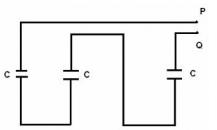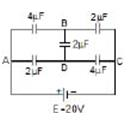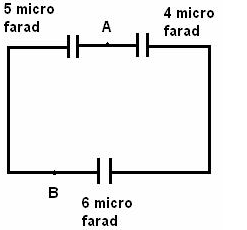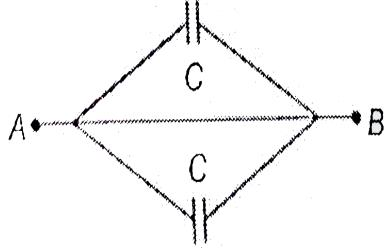Courses

# Test: Combination Of Capacitors

## 10 Questions MCQ Test Physics Class 12 | Test: Combination Of Capacitors

Description
This mock test of Test: Combination Of Capacitors for Class 12 helps you for every Class 12 entrance exam. This contains 10 Multiple Choice Questions for Class 12 Test: Combination Of Capacitors (mcq) to study with solutions a complete question bank. The solved questions answers in this Test: Combination Of Capacitors quiz give you a good mix of easy questions and tough questions. Class 12 students definitely take this Test: Combination Of Capacitors exercise for a better result in the exam. You can find other Test: Combination Of Capacitors extra questions, long questions & short questions for Class 12 on EduRev as well by searching above.
QUESTION: 1

### Here is a combination of three identical capacitors. If resultant capacitance is 1 μƒ, calculate capacitance of each capacitor.Solution:

Combination of Capacitors in Series
C(effective) = C/3 = 1

QUESTION: 2

Solution:

Definition

QUESTION: 3

### If two spheres of different radii have equal charge, then the potential will be

Solution:

When equal charges are given to two spheres of different radii, the potential will be more or the smaller sphere as per the equation, Potential = Charge / Radius.
Since potential is inversely proportional to radius, the smaller radius will have higher potential and vice versa.

*Multiple options can be correct
QUESTION: 4

The potential of the point B and D areSolution:
QUESTION: 5

Three different capacitors are connected in series, then:

Solution:
QUESTION: 6

Calculate the equivalent capacitance for the following combination between points A and BSolution:

4μf and 6μf are in series (right side of AB) so 6x(4/6)+4=24/10μf
And now 5 μf and the resulting of above two are in parallel (as on different sides of AB) so 5+(24/10) =50+(24/10)=74/10=37/5μf

QUESTION: 7

There are three capacitors with equal capacitance. In series combination, they have a net capacitance of Cand in parallel combination, a net capacitance of C2.What will be the value of C1 C2?​

Solution:

For series combination,
1/C1=(1/C)+(1/C)+(1/C) [ Since all capacitors have equal capacitances]=3/C Or
C1=C/3−−−(i)
For parallel combination,
C2=C+C+C=3C−−−(ii)
Now,C1/C2=(C/3)/3C=(C/3)×(1/3C)=1/9

QUESTION: 8

Two capacitors of 20 μƒ and 30 μƒ are connected in series to a battery of 40V. Calculate charge on each capacitor.​

Solution:

C1= 20×10µf

and C2= 30×10µf

in series Ceq = C1C2/(C1+C2)

Ceq = 20×10^(-6)×30×10^(-6)/20×10^(-6)+30^×10(-6)

Ceq= 12×10^(-6)f

As we know that Q = CV

Putting the values of C and V= 40V, we get

Q = (12 * 10^-6) * 40

= 480µC

QUESTION: 9

Two capacitors of equal capacity are first connected in parallel and then in series. The ratio of the total capacities in the two cases will be

Solution:

Third capacitor is short-circuited as its both ends are connected to B. Equivalent circuit isCeq=C+C=2C

QUESTION: 10

When two capacitors C1 and C2 are connected in series and parallel, their equivalent capacitances comes out to be 3μƒ and 16μƒ respectively. Calculate values of C1 and C2.

Solution: